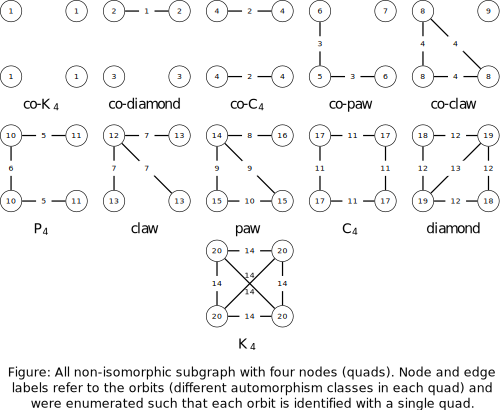# Introduction to oaqc

This package provides an efficient algorithm to calculate for a given graph the orbit-aware quad census. More precisely the frequency distribution of all induced and non-induced non-isomorphic four node subgraphs, i.e. quads, on a node and edge level; see the figure below for the relation between orbit and quad.## Input

The input can either be

• Edge-List (matrix or data.frame)
• Graph Object ('igraph')

Despite the input format the graph should not contain loops or multi-edges and the vertex indices have to lie in range $$[0,n-1)$$ with $$n$$ denoting the number of vertices in the graph. Note that if the smallest index is $$1$$ the algorithm will create an isolated vertex with index $$0$$.

## Calculating the orbit-aware quad census

The following code exemplifies the use of this package.

library(oaqc)
### k4, pure R
k4 <- data.frame(
source = c(0, 0, 0, 1, 1, 2),
target = c(1, 2, 3, 2, 3, 3)
)
k4orbits <- oaqc(k4, non_ind_freq = F, file = "")
# print(k4orbits)


In order to calculate the non-induced frequencies as well just set the corresponding flag to TRUE.

Since the orbit-aware frequencies can be rather large integers, which can cause some problems with R, the results can be directly written to a file.

## Result

The results of the, e.g., induced frequencies of the nodes in orbit 10 can be accessed in the following way.

print(k4orbits\$n_orbits_ind[,10])

##  0 0 0 0